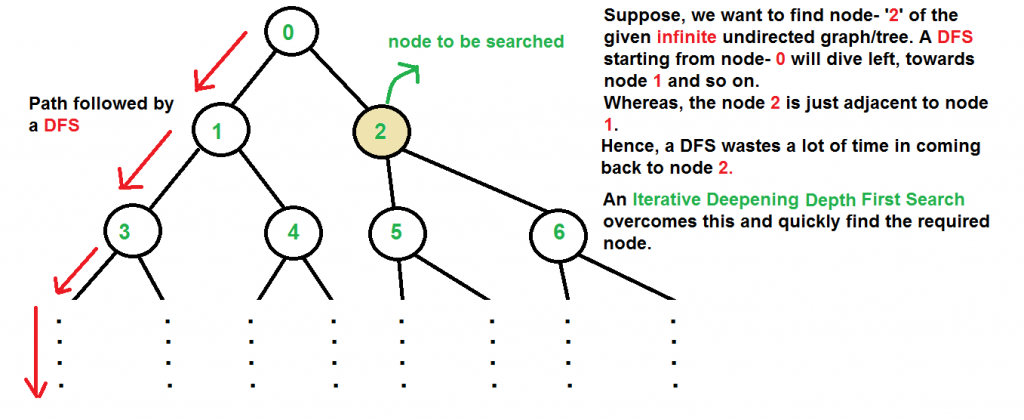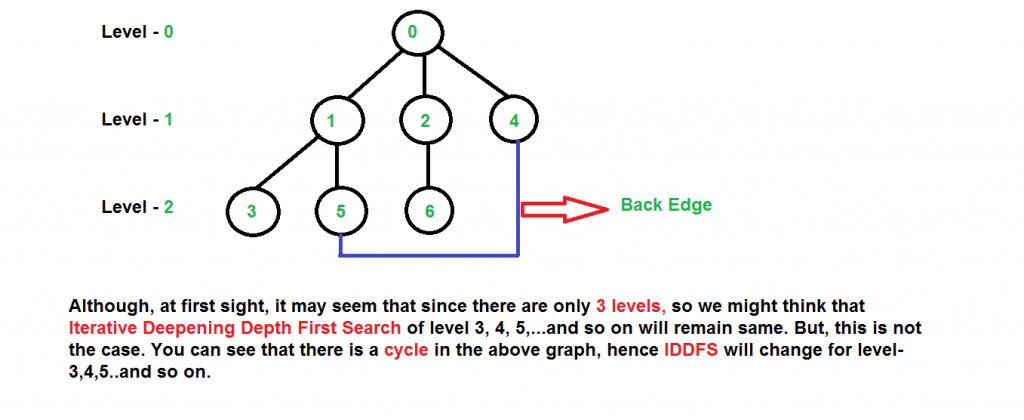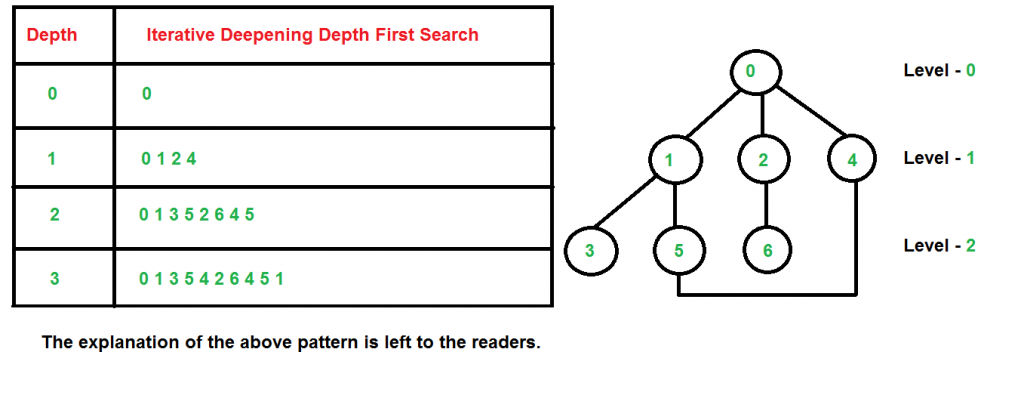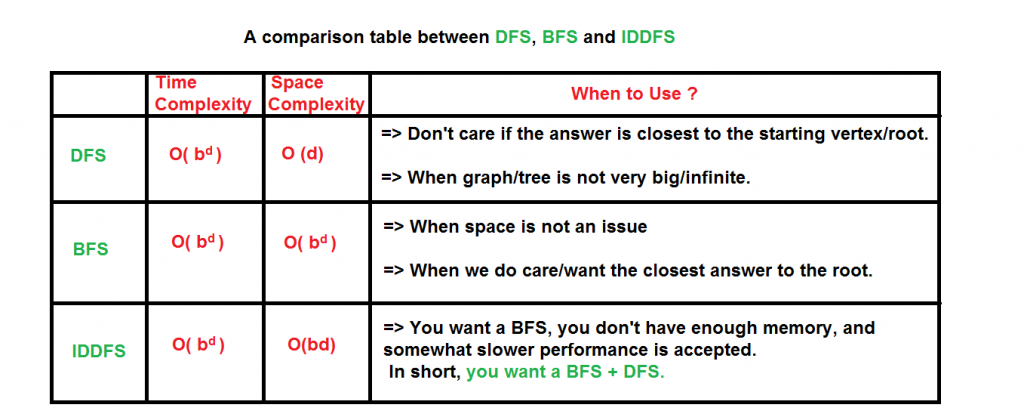Skip to content
Related Articles
Iterative Deepening Search(IDS) or Iterative Deepening Depth First Search(IDDFS)
• Difficulty Level : Medium
• Last Updated : 22 Dec, 2016

There are two common ways to traverse a graph, BFS and DFS. Considering a Tree (or Graph) of huge height and width, both BFS and DFS are not very efficient due to following reasons.

1. DFS first traverses nodes going through one adjacent of root, then next adjacent. The problem with this approach is, if there is a node close to root, but not in first few subtrees explored by DFS, then DFS reaches that node very late. Also, DFS may not find shortest path to a node (in terms of number of edges).2. BFS goes level by level, but requires more space. The space required by DFS is O(d) where d is depth of tree, but space required by BFS is O(n) where n is number of nodes in tree (Why? Note that the last level of tree can have around n/2 nodes and second last level n/4 nodes and in BFS we need to have every level one by one in queue).

IDDFS combines depth-first search’s space-efficiency and breadth-first search’s fast search (for nodes closer to root).

How does IDDFS work?
IDDFS calls DFS for different depths starting from an initial value. In every call, DFS is restricted from going beyond given depth. So basically we do DFS in a BFS fashion.

Algorithm:

```// Returns true if target is reachable from
// src within max_depth
bool IDDFS(src, target, max_depth)
for limit from 0 to max_depth
if DLS(src, target, limit) == true
return true
return false

bool DLS(src, target, limit)
if (src == target)
return true;

// If reached the maximum depth,
// stop recursing.
if (limit <= 0)
return false;

foreach adjacent i of src
if DLS(i, target, limit?1)
return true

return false```

An important thing to note is, we visit top level nodes multiple times. The last (or max depth) level is visited once, second last level is visited twice, and so on. It may seem expensive, but it turns out to be not so costly, since in a tree most of the nodes are in the bottom level. So it does not matter much if the upper levels are visited multiple times.

Below is implementation of above algorithm

## C/C++

 `// C++ program to search if a target node is reachable from``// a source with given max depth.``#include``using` `namespace` `std;`` ` `// Graph class represents a directed graph using adjacency``// list representation.``class` `Graph``{``    ``int` `V;    ``// No. of vertices`` ` `    ``// Pointer to an array containing``    ``// adjacency lists``    ``list<``int``> *adj;`` ` `    ``// A function used by IDDFS``    ``bool` `DLS(``int` `v, ``int` `target, ``int` `limit);`` ` `public``:``    ``Graph(``int` `V);   ``// Constructor``    ``void` `addEdge(``int` `v, ``int` `w);`` ` `    ``// IDDFS traversal of the vertices reachable from v``    ``bool` `IDDFS(``int` `v, ``int` `target, ``int` `max_depth);``};`` ` `Graph::Graph(``int` `V)``{``    ``this``->V = V;``    ``adj = ``new` `list<``int``>[V];``}`` ` `void` `Graph::addEdge(``int` `v, ``int` `w)``{``    ``adj[v].push_back(w); ``// Add w to v’s list.``}`` ` `// A function to perform a Depth-Limited search``// from given source 'src'``bool` `Graph::DLS(``int` `src, ``int` `target, ``int` `limit)``{``    ``if` `(src == target)``        ``return` `true``;`` ` `    ``// If reached the maximum depth, stop recursing.``    ``if` `(limit <= 0)``        ``return` `false``;`` ` `    ``// Recur for all the vertices adjacent to source vertex``    ``for` `(``auto` `i = adj[src].begin(); i != adj[src].end(); ++i)``       ``if` `(DLS(*i, target, limit-1) == ``true``)``          ``return` `true``;`` ` `     ``return` `false``;``}`` ` `// IDDFS to search if target is reachable from v.``// It uses recursive DFSUtil().``bool` `Graph::IDDFS(``int` `src, ``int` `target, ``int` `max_depth)``{``    ``// Repeatedly depth-limit search till the``    ``// maximum depth.``    ``for` `(``int` `i = 0; i <= max_depth; i++)``       ``if` `(DLS(src, target, i) == ``true``)``          ``return` `true``;`` ` `    ``return` `false``;``}`` ` `// Driver code``int` `main()``{``    ``// Let us create a Directed graph with 7 nodes``    ``Graph g(7);``    ``g.addEdge(0, 1);``    ``g.addEdge(0, 2);``    ``g.addEdge(1, 3);``    ``g.addEdge(1, 4);``    ``g.addEdge(2, 5);``    ``g.addEdge(2, 6);`` ` `    ``int` `target = 6, maxDepth = 3, src = 0;``    ``if` `(g.IDDFS(src, target, maxDepth) == ``true``)``        ``cout << ``"Target is reachable from source "``                ``"within max depth"``;``    ``else``        ``cout << ``"Target is NOT reachable from source "``                ``"within max depth"``;``    ``return` `0;``}`

## Python

 `# Python program to print DFS traversal from a given``# given graph``from` `collections ``import` `defaultdict`` ` `# This class represents a directed graph using adjacency``# list representation``class` `Graph:`` ` `    ``def` `__init__(``self``,vertices):`` ` `        ``# No. of vertices``        ``self``.V ``=` `vertices`` ` `        ``# default dictionary to store graph``        ``self``.graph ``=` `defaultdict(``list``)`` ` `    ``# function to add an edge to graph``    ``def` `addEdge(``self``,u,v):``        ``self``.graph[u].append(v)`` ` `    ``# A function to perform a Depth-Limited search``    ``# from given source 'src'``    ``def` `DLS(``self``,src,target,maxDepth):`` ` `        ``if` `src ``=``=` `target : ``return` `True`` ` `        ``# If reached the maximum depth, stop recursing.``        ``if` `maxDepth <``=` `0` `: ``return` `False`` ` `        ``# Recur for all the vertices adjacent to this vertex``        ``for` `i ``in` `self``.graph[src]:``                ``if``(``self``.DLS(i,target,maxDepth``-``1``)):``                    ``return` `True``        ``return` `False`` ` `    ``# IDDFS to search if target is reachable from v.``    ``# It uses recursive DLS()``    ``def` `IDDFS(``self``,src, target, maxDepth):`` ` `        ``# Repeatedly depth-limit search till the``        ``# maximum depth``        ``for` `i ``in` `range``(maxDepth):``            ``if` `(``self``.DLS(src, target, i)):``                ``return` `True``        ``return` `False`` ` `# Create a graph given in the above diagram``g ``=` `Graph (``7``);``g.addEdge(``0``, ``1``)``g.addEdge(``0``, ``2``)``g.addEdge(``1``, ``3``)``g.addEdge(``1``, ``4``)``g.addEdge(``2``, ``5``)``g.addEdge(``2``, ``6``)`` ` `target ``=` `6``; maxDepth ``=` `3``; src ``=` `0`` ` `if` `g.IDDFS(src, target, maxDepth) ``=``=` `True``:``    ``print` `(``"Target is reachable from source "` `+``        ``"within max depth"``)``else` `:``    ``print` `(``"Target is NOT reachable from source "` `+``        ``"within max depth"``)`` ` `# This code is contributed by Neelam Pandey`

Output :
`Target is reachable from source within max depth`

Illustration:
There can be two cases-
a) When the graph has no cycle: This case is simple. We can DFS multiple times with different height limits.

b) When the graph has cycles. This is interesting as there is no visited flag in IDDFS.Time Complexity: Suppose we have a tree having branching factor ‘b’ (number of children of each node), and its depth ‘d’, i.e., there are bd nodes.

In an iterative deepening search, the nodes on the bottom level are expanded once, those on the next to bottom level are expanded twice, and so on, up to the root of the search tree, which is expanded d+1 times. So the total number of expansions in an iterative deepening search is-

``` (d)b + (d-1)b2 + .... + 3bd-2 + 2bd-1 + bd

That is,
Summation[(d + 1 - i) bi], from i = 0 to i = d
Which is same as O(bd)
```

After evaluating the above expression, we find that asymptotically IDDFS takes the same time as that of DFS and BFS, but it is indeed slower than both of them as it has a higher constant factor in its time complexity expression.

IDDFS is best suited for a complete infinite treeThis article is contributed by Rachit Belwariar. If you like GeeksforGeeks and would like to contribute, you can also write an article and mail your article to contribute@geeksforgeeks.org. See your article appearing on the GeeksforGeeks main page and help other Geeks.

Please write comments if you find anything incorrect, or you want to share more information about the topic discussed above

Attention reader! Don’t stop learning now. Get hold of all the important DSA concepts with the DSA Self Paced Course at a student-friendly price and become industry ready.  To complete your preparation from learning a language to DS Algo and many more,  please refer Complete Interview Preparation Course.

In case you wish to attend live classes with industry experts, please refer Geeks Classes Live

My Personal Notes arrow_drop_up